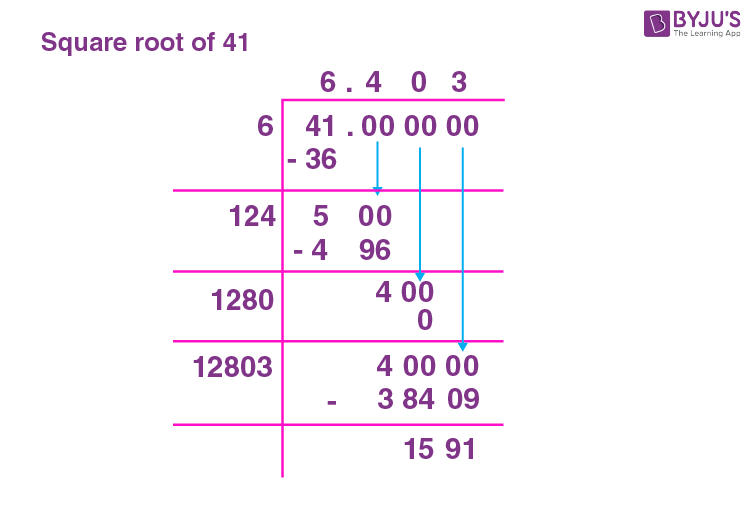# Square Root of 41

The square root of 41 is irrational. The approximate value of the square root of 41 is 6.4. When 6.4 is multiplied by 6.4, the product is 40.96, which is approximately equal to 41. Since 6.4 is multiplied by itself, 6.4 is the nearest square root of 41. In general, when an integer “p” is multiplied with the same integer “p”, the resultant “z” is called the perfect square and the integer “p” is its square root. i.e., If ± p × ± p = z, then p is the square root of z.

The given number 41 lies between 36 and 49, where 36 is obtained by the square of 6 ( ±6 × ±6 = 36) and 49 is obtained by multiplying 7 with 7. (±7 × ±7 = 49).

This means, that 41 is not a perfect square and it does not have a perfect square root. Hence, the square root of 41, √41 is an irrational number. You can refer to Finding Square root with Example for details.

Note the Following:

• The Square root of 41 = √41 = 2√41, where ‘√’ is the radical, 41 is the radicand and 2 is the index
• Exponential Form of Square root of 41 = 411/2.
• Solution for √41 ≈ 6.4
• The square root of 41 is Irrational = True.

## What is the Square root of 41?

The square root of 41 is 6.4, as the square root of 41 is irrational.

 √41 = irrational =6.4

## How to Find the Square root of 41?

There are three methods to find the Square root of 41

• Prime Factorisation method
• Long Division method
• Repeated Subtraction method

### Square root of 41 by Prime Factorisation Method

In the prime factorization method, the following steps are followed.

1. Divide 41 with prime factors. Since 41 is a prime number, there are no other factors than 1 and 41.

Hence the square root of 41 is √41, which is irrational.

The given number, 41, will be expressed as;

 41 41 × 1

### Square root of 41 by Long Division Method

In the Long Division method, for 41, divide 41 by selecting a divisor such that d × d is less than or equal to 41. If d = 41, then d is the perfect square root of 41, else it is irrational.

Choosing d from the list would be,

the squares are

6 × 6 = 36

7 × 7 = 49

No further division is possible and the remainder is non-zero, which is 5.Hence 41 is not a perfect square. Therefore the square root of 41 is an irrational number.

### Square root of 41 by Repeated Subtraction Method.

In the repeated Subtraction method, 41 is subtracted by odd numbers starting with 1, expecting the difference to become zero at some point of the subtraction process. If zero is obtained, then 41 is a perfect square. Else 41 is not a perfect square.

The table below shows repeated subtraction for the given number 41.

 Step 1 41 – 1 = 40 Step 2 40 – 3 = 37 Step 3 37 – 5 = 32 Step 4 32 – 7 = 25 Step 5 25 – 9 = 16 Step 6 16 – 11 = 5 Step 7 5 – 13 = -8

Since the difference does not become zero but -8, 41 is not a perfect square number and hence it does not have a perfect square root. The root lies between the numbers 6 and 7, resulting in a decimal root.

## Video Lessons

### Visualising square roots### Finding Square roots## Solved Examples

1. What needs to be added to 41, to make it a perfect square?

8 needs to be added to 41 to make it 49, which is a perfect square number. The square root is 7.

2. What is the square of 41?

41 square is 1681.

## Frequently Asked Questions on Square root of 41

### What is the Square root of 41?

The Square root of 41 is 6.4

### 41 is a perfect square number. True or False?

False. 41 is not a perfect square number.

### What are the factors of 41?

Since 41 is a prime number, the factors of 41 are 1 and 41.

### Simplify √41?

√41 = √(1 × 41). No further simplification is possible.

### The square root of 41 is a rational number. True or False?

False. The square root of 41 is not a rational number.